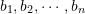### Python Lab Tutorials: Lab 7

The following data provides the number of trucks with a particular weight at each hour of the day on one of the busy US highways. The data is provided as (h_i,N_i), where h_i is the hour and N_i is the number of trucks:
(1,16), (2,12), (3,14), (4,8), (5,24), (6,92), (7,311), (8,243), (9,558), (10,644), (11,768), (12,838), (13,911), (14,897), (15,853), (16,860), (17,853), (18,875), (19,673), (20,378), (21,207), (22,142), (23,108), (24,62).

• Graph the data.
• Use Sympy’s “interpolate” to find the interpolating polynomial. Then use Scipy’s “interp1d” to find the piecewise polynomial of order 0, the piecewise polynomial of order 1, the piecewise polynomial of order 2, and the piecewise polynomial of order 3 that fit the data.
• Plot the graphs of the following:
The interpolating polynomial overlapped with the data
The piecewise polynomial of order 0 overlapped with the data
The piecewise polynomial of order 1 overlapped with the data
The piecewise polynomial of order 2 overlapped with the data
The piecewise polynomial of order 3 overlapped with the data
• Use the interpolating polynomial, the piecewise polynomial of order 0, the piecewise polynomial of order 1, the piecewise polynomial of order 2, and the piecewise polynomial of order 3 to predict the number of trucks on the highway at t=4.5 hr and t=13.5 hr.
• Remove the data point (19,673) and use Python to find the interpolating polynomial, the piecewise polynomial of order 0, the piecewise polynomial of order 1, the piecewise polynomial of order 2, and the piecewise polynomial of order 3 that fit the data.
• Plot the interpolating polynomial and the piecewise polynomials overlapping the original data.
• Use these new equations to predict the number of trucks at t=19 hr. Comment on the difference between the prediction and the actual recorded value.
• (bonus worth 1% to be submitted by email ):
Provide a Python procedure whose input is the table of data points and whose output is the vector of the coefficientsof the Newton Interpolating Polynomial.